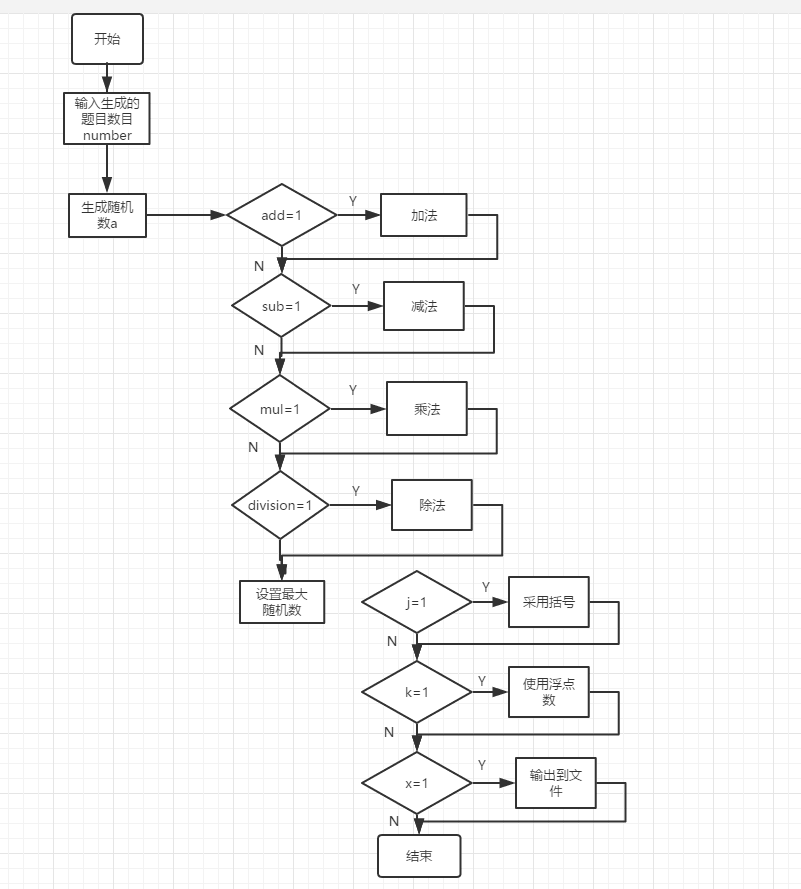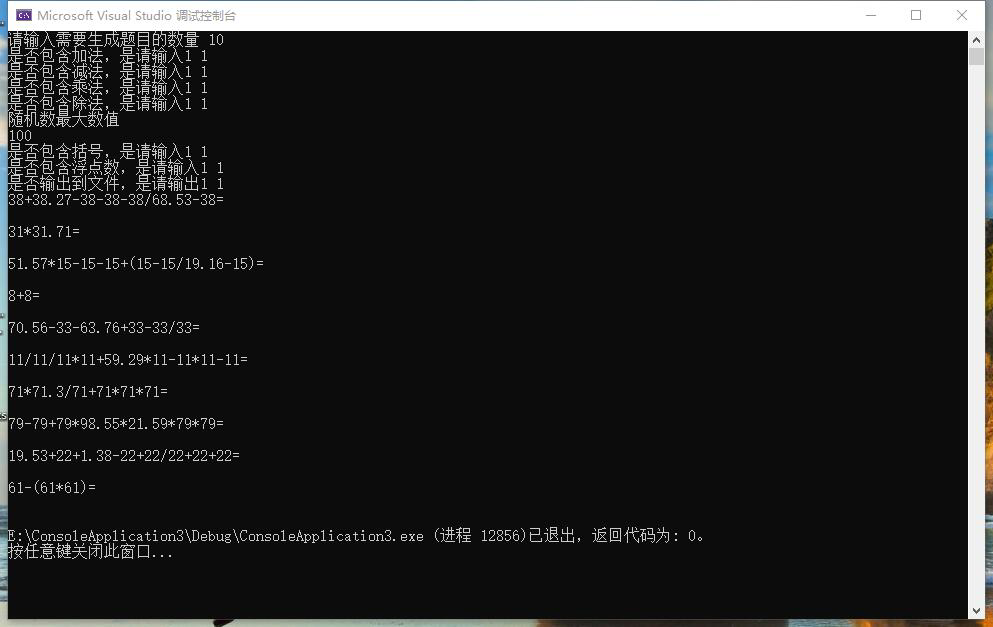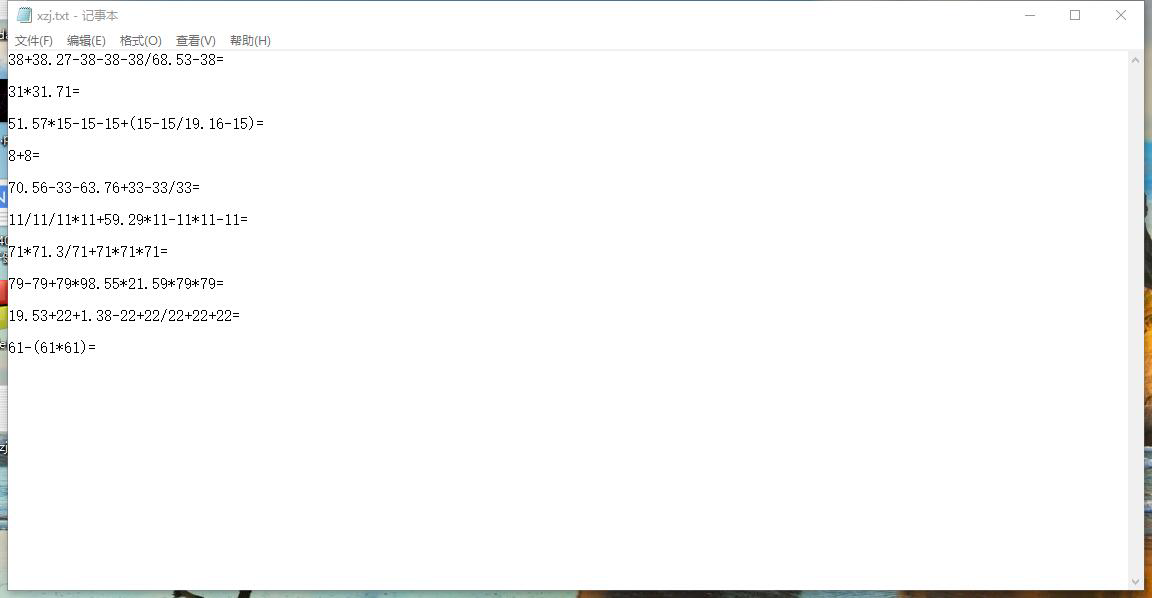# 软件工程结对编程第二次作业

我们在刚开始上课的时候介绍过一个小学四则运算自动生成程序的例子，请实现它，要求：
能够自动生成四则运算练习题
可以定制题目数量
用户可以选择运算符
用户设置最大数（如十以内、百以内等）
用户选择是否有括号、是否有小数
用户选择输出方式（如输出到文件、打印机等）
最好能提供图形用户界面（根据自己能力选做，以完成上述功能为主）

[conding地址]:(https://git.dev.tencent.com/dtid_b9d45a3fda189455/jieduibianchengdiercizuoye.git)int main()
{
ofile.open("E:\xzj.txt");
int number;
int a, b, c, d, sum, i, l, j, x,k;
cout << "请输入需要生成题目的数量" << ' ';
cin >> number;
cout << "是否包含加法，是请输入1" << ' ';
cin >> a;
if (a == 1)
{
}
cout << "是否包含减法，是请输入1" << ' ';
cin >> b;
if (b == 1)
{
sub = 1;
}
cout << "是否包含乘法，是请输入1" << ' ';
cin >> c;
if (c == 1)
{
mul = 1;
}
cout << "是否包含除法，是请输入1" << ' ';
cin >> d;
if (d == 1)
{
division = 1;
}
cout << "随机数最大数值" << endl;
cin >> l;
cout << "是否包含括号，是请输入1" << ' ';
cin >> j;
cout << "是否包含浮点数，是请输入1" << ' ';
cin >> x;
cout << "是否输出到文件，是请输出1" << ' ';
cin >> k;
if (j == 1)
{
parentheses = 1;
}
if (x == 1)
{
FloatingpointNumbers = 1;
}
sum = Generationofarray(Add, sub, mul, division);
if (l <= 101)
{
arithmetic(number, sum, 101);
}
ofile.close();
return 0;
}posted @ 2019-05-06 19:51  YELLOWCARD  阅读(147)  评论(0编辑  收藏  举报